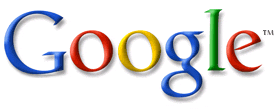# Html Tips and Tricks

## Thursday, February 02, 2006

### Images Clickable in Different Areas

Before, looking at these HTML tips and tricks, be sure to know the tips on links and images.

Look at the following:It's one image (the Google logo). However, try clicking on different parts of the image. It leads you to different web pages. The code below made the HTML tip:

<area shape=rect coords=68,0,161,109 href="http://en.wikipedia.org/wiki/O">
<area shape=rect coords=161,0,201,109 href="http://en.wikipedia.org/wiki/G">
<area shape=rect coords=201,0,227,109 href="http://en.wikipedia.org/wiki/L">
<area shape=rect coords=227,0,275,109 href="http://en.wikipedia.org/wiki/E">
</map>

The first line inserts an image, called logo.gif. usemap=#GoogleImageMap calls the clickable areas GoogleImageMap. You can change the name GoogleImageMap to any other name you want, like GoogleLetters. Then, you have the 'Image Map':

<area shape=rect coords=0,0,68,109 href="http://en.wikipedia.org/wiki/G">
<area shape=rect coords=68,0,161,109 href="http://en.wikipedia.org/wiki/O">
<area shape=rect coords=161,0,201,109 href="http://en.wikipedia.org/wiki/G">
<area shape=rect coords=201,0,227,109 href="http://en.wikipedia.org/wiki/L">
<area shape=rect coords=227,0,275,109 href="http://en.wikipedia.org/wiki/E">
</map>

Make sure you change GoogleImageMap to the name of your image map. The italicized numbers stand for the right, top, left and bottom edges of each clickable rectangle area. The E letter, for example, has 227 as the right edge, 0 as the top edge, 275 as the left edge and 109 as the bottom edge. To find the coordinates of a clickable rectangle in any picture, you can copy and paste the picture into Paint. (See Note below to find out how to find out the numbers.) The href="http://..." tells which web page the viewer will go to when he/she clicks on the rectangle.

Try clicking everywhere around the E. You will see that in the rectangle defined by the coordinates 227,0,275,109 goes to http://en.wikipedia.org/wiki/E.Remember, you can add and delete areas in order to add or remove clickable rectangles:

<area shape=rect coords=201,0,275,109 href="http://en.wikipedia.org/wiki/Le">
</map>

Above I have removed the rectangles for O,G,L,E but added a rectangle for Le. Here is the result:These tips and tricks can add a few touches of proffesionalism to the pictures on your web page! Remember to check out a few other tips in this web site!

NOTE: To find out the numbers next to coords that define a rectangle, copy and paste the image into Paint. Move your mouse across the image and look at the bottom left corner of Paint. You will see two numbers (for example, 47,56). 47 shows how far left your mouse is, and 56 shows how for down your mouse is. To find out the first two numbers you need to put next to coords=, move your mouse to the upper left of the rectangle you want, then look at the two numbers at the corner. To find out the last two numbers, move your mouse to the lower right of the rectangle, then look at the two numbers at the corner.

NEED EXTRA HELP? HAVE A SUGGESTION? HAVE SOMETHING TO ADD? PLEASE POST COMMENTS WHILE LOOKING AT ALL THESE USEFUL HTML TIPS AND TRICKS. THANK YOU!